### Convert numbers to minutes

If you have a series of values like this:

12.5
9.3
10.7

and want to convert this numbers to a minutes/seconds format you can use this formula:

=A1/(24*60)

This assumes that the values below are on cells A1:A3 and this formula is placed on cell B1 and copied down. You need to format the cells B1:B3 as Custom mm:ss to see this:

12:30
09:18
10:42

### Multiply range of values by a value

One of the most common tasks that we need to do in Excel is multiply a range of value on a sheet by a specific value, say, multiply a range of values by 100. This can be done very easily by following this steps. Say you have this sheet:In cell A5 you should put the value that you want to use to multiply the values on cells A1:D3. Now on cell A5 make a Ctrl+C (Copy) and select the cells A1:D3. Right-click on top of the range and select Paste Special, on Paste options select the Values and on Operation select Multiply. Click OK. Now your sheet should look like this:If instead of multipying the values by 100, you Add, Substract or Divide the values by the value that you select on another cell (in this case I used the cell A5).

### Get row number from a match

Excel has a function to locate a value on a range of cells and return the number of the row where the value is found, it’s the MATCH() function. The syntax is like this:

MATCH(lookup_value,lookup_array,match_type)

lookup_value is the value that we want to look for and lookup_array is the range of cells where we want to search. match_type can have 3 values:
1 - finds the largest value that is less than or equal to lookup_value. Lookup_array must be placed in ascending order;
0 - finds the first value that is exactly equal to lookup_value
-1 - finds the smallest value that is greater than or equal to lookup_value. Lookup_array must be placed in descending order.
If match_type is omitted, it will assume to be 1.
Here’s an example. We have the following table:We want to find the name Mary on the range of A2:A6. So we should use this formula:

=MATCH("Mary",A2:A6,0)

This will return 2 because the name Mary can be found on cell A2.

### Excel Charts - Add totals labels to Stacked Column chart

Let's say that I have the following table of data: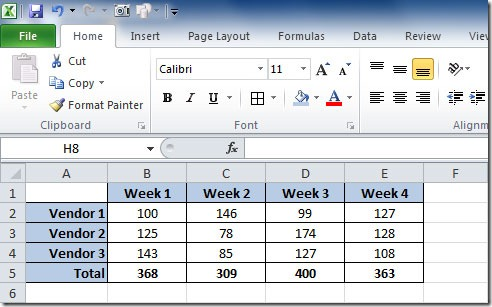I can make a Stacked Column chart with 3 series, one for each Vendor. The chart will look like this: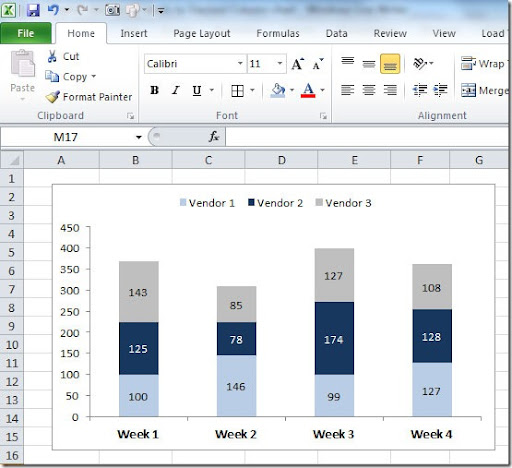But I want to display on the top of each bar, the total for the Week (the sum of each Vendor). To do that, I need to add a 4th series to the chart. For that I select the chart and right-click on top of it and select Source Data. On the Series tab, on the Series, click on the Add button to add a new series. For the Values range, select the cells where the values of Total are on our table. For the Name, select the cell where we have "Total". Click OK and you will get a chart like this: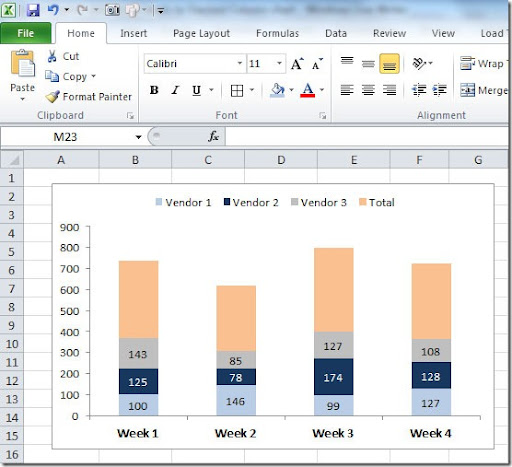Select this new series on the chart, right click and choose Format Data Series. On the Data Labels tab, Label Contains mark Value. Then on the Patterns tab put Border-None and Area-None. Click OK. Now select the labels, right click on them, choose Format Data Labels and on the Alignment tab, Label Position choose Inside Base. Click OK. You need to adjust the scale of your chart so that it looks like the original one. In this case, I changed Maximum value to 450 instead of Automatic. As you can see, the “Total” series name still shows up on the Legend. You need to select the Total legend and delete. The final result will be this something like this: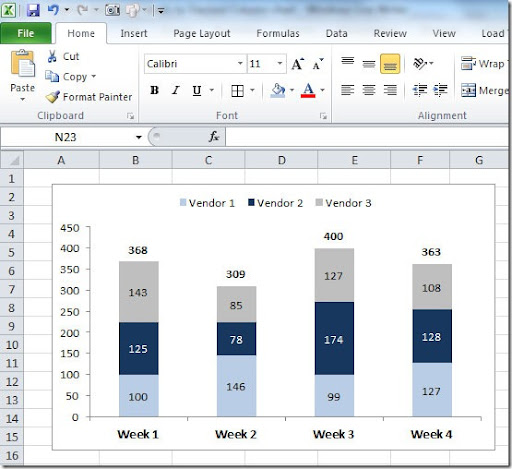Hope this helps you create better charts in Excel!

### VBA – Define dynamic ranges

In VBA code we can define a dynamic range by checking the last row that has data on it. this can be defined in various ways. Here is a simple way of doing it:

Dim rng As Range
rng = Range("A12:C" & Worksheets("Sheet1").Range("C65535").End(xlUp).Row + 1)

This will get us the range A12:C200 if C200 is the last used cell in column C.
You can also define a range that gets all of the columns in row 1 that are used as column headers, like this:

Dim ws As Worksheet
Dim rng As RangeSet
ws = ActiveSheetSet
rng = Intersect(ws.Rows(1), ws.UsedRange)

This will get us the range of A1:D4 if columns A, B, C and D have data on it.

### VBA – Get font color Function

When we have colors on our sheets data and want, for instance, to count how many “red” words we have on our sheet, that is not possible because there is no formula in Excel to check for font colors. Instead we can create our own VBA Function to get the font color. It’s a very simple code. You have to insert it on a VBA module on your sheet.

Function GetFontColor(ByVal Target As Range) As Object
GetFontColor = Target.Font.ColorIndex
End Function

Then you can use it on your sheet like this:

GETFONTCOLOR(A2)

Below is an example on how you can use this function. In column C we put the font color of text in column A.To count the number of “red” words in column A we can simple to this:

COUNTIF(C2:C9,3)

“3” in the formula refers to the color red.

### Add-in – Save Excel 2007 file as Pdf

Many times we need to send our Excel files to someone but we don’t want them to be able to edit our files. One of the ways that we can use to do that is to save the Excel sheet as a PDF file. With Office 2007 we don’t need a third party tool to convert our sheets, we can install an Add-in from Microsoft that can be found on the link below:

This Add-in allows you to export not only Excel sheets but also other Office 2007 programs. The sheets can be exported either to PDF or XPS format. It also allows to send as an email attachment in this formats.

### VBA – Combine sheets data into one sheet

I have a Workbook with multiple sheets, all with the same configuration, with headers on row 1 and data starting from row 2.
I want to combine the data from all the sheets into one single sheet called “Target”. I have to go through all the sheets and check what is the last row and the last column with data to define the range that I’m going to copy to the Target sheet. I think that the code is all well commented for you to understand how this is done. This is a simple example how to combine data from multiple sheets into one single sheet. This can be done other ways or can be more complex with sheets with different columns or so. This will be handled in future articles.

`Sub CombineSheets()     'This macro will copy all rows from the first sheet     '(including headers)     'and on the next sheets will copy only the data     '(starting on row 2)     Dim i As Integer     Dim j As Long     Dim SheetCnt As Integer     Dim lstRow1 As Long    Dim lstRow2 As Long    Dim lstCol As Integer     Dim ws1 As Worksheet     With Application         .DisplayAlerts = False         .EnableEvents = False         .ScreenUpdating = False     End With     On Error Resume Next     'Delete the Target Sheet on the document (in case it exists)     Sheets("Target").Delete     'Count the number of sheets on the Workbook     SheetCnt = Worksheets.Count     'Add the Target Sheet     Sheets.Add after:=Worksheets(SheetCnt)     ActiveSheet.Name = "Target"     Set ws1 = Sheets("Target")     lstRow2 = 1     'Define the row where to start copying     '(first sheet will be row 1 to include headers)     j = 1     'Combine the sheets     For i = 1 To SheetCnt         Worksheets(i).Select         'check what is the last column with data         lstCol = ActiveSheet.Cells(1, Activesheet.Columns.Count).End(xlToLeft).Column         'check what is the last row with data         lstRow1 = ActiveSheet.Cells(activesheet.rows.count, "A").End(xlUp).Row        'Define the range to copy         Range("A" & j, Cells(lstRow1, lstCol)).Select         'Copy the data         Selection.Copy         ws1.Range("A" & lstRow2).PasteSpecial         Application.CutCopyMode = False         'Define the new last row on the Target sheet         lstRow2 = ws1.Cells(65536, "A").End(xlUp).Row + 1         'Define the row where to start copying         '(2nd sheet onwards will be row 2 to only get data)         j = 2     Next     With Application         .DisplayAlerts = True         .EnableEvents = True         .ScreenUpdating = True     End With     Sheets("Target").Select     Cells.EntireColumn.AutoFit     Range("A1").Select End Sub`

### Excel Charts – Do not show empty dates

When we create a new chart in Excel, if the X axis labels are dates, Excel will assume “Automatic” on the Primary axis Category. Please check image below.On this example I have dates 1, 2, 5, 6 and 7 of October. Only this dates have data on it.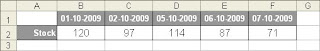With the option Category X axis set to Automatic, Excel will produce a chart like Chart 1: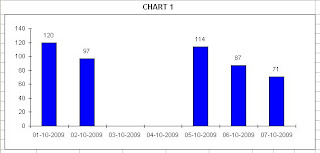As you can see, Excel has added the dates that where missing between 2 and 5 of October. This dates are not a part of our original table and don’t have value to be charted so I don’t want to display them. This happens because Excel assumes that this is a Time-scale category chart. To remove this dates, go to Chart Options and set the Primary Axis-Category X axis to “Category”. This way we get a chart like Chart 2: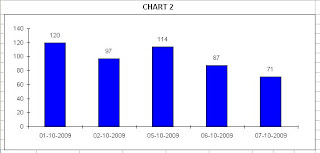### Splitting a string

There are numerous occasions when we need to split a string into pieces. Depending on the type of string that we are handling we can do it using many different techniques. Here I’m going to show you one way of doing a simple string split. On column A we have the strings that we want to split. We want to get the text that is before the “,” character into column B.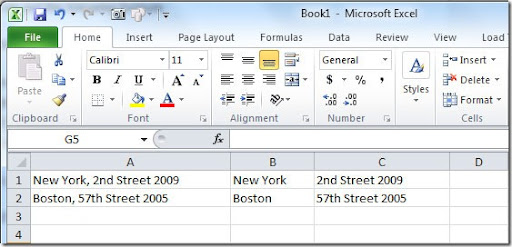So in column B we can put the following formula:

=TRIM(LEFT(A1,FIND(",",A1)-1))

Here I use 3 Excel functions: TRIM(), to remove the spaces on the beginning and on the end of the string that we get; LEFT() to get the string to the left of the “splitter”, in this case is the , character and FIND() to find the position of the “splitter” character. I remove 1 from the position that FIND() returns so that I don’t get the “,” character.

In column C we can put the following formula to the the text that is on the right of the “,” character:

=TRIM(RIGHT(A1,LEN(A1)-FIND(",",A1)))

Here, instead of the LEFT() function, I use the RIGHT() because I want to get the text on the right of the “splitter” character. I use the LEN() function to get the number of character that the string on column A has so that I can subtract the position where the FIND() function finds the “splitter” character. This way I get the number of characters for the function RIGHT() to return, counting from the end of the string in column A. Example: the string in cell A1 has a LEN(A1) of 25 characters. The “splitter” character is found on position 9 of the string. So my formula will return on cell C1 (25-9)=16 characters counting from the right of string in cell A1, the result will be “2nd Street 2009”.

### VBA - Convert emails to hyperlinks

On a sheet column I have email addresses and I want to convert them to hyperlinks so that when I click them, I open my email client and send and email to that address. I can use a macro to change the content of that cell to a hyperlink. Here’s the code for that:

Dim rng As Range
Dim cell As Range

'Define here the range where you have your emails values
rng = Range("B2:B100")

For Each cell In rng
'If the cell is blank, ignore it
If cell.Value <> "" Then
End If
Next cell

End Sub

### Create random combinations in Excel

Imagine that you need to create random combinations of letters in Excel. In this example I want to create a 3 letters random combinations list. I want the result to be like this:

 TEX JYY QCX CDH NTW

To get this kind of combinations I used the following formula:

=CHAR(RANDBETWEEN(65,90))&CHAR(RANDBETWEEN(65,90))&CHAR(RANDBETWEEN(65,90))

I’ve used the RANDBETWEEN() function to generate values between 65 and 90 because they are the ANSI codes of letters A to Z. The RANDBETWEEM() function has the following arguments:

RANDBETWEEN(bottom,top)

bottom is the smallest integer that the function will return and top is the highest.

After the generation of the random number, I use CHAR() function to return the corresponding character from the ANSI table of characters. This function has the following syntax:

CHAR(number)

number is a number between 1 and 255 that specifies which character we want to return.

On my formula, combining 3 times the formula CHAR(RANDBETWEEN(65,90) I get a combination of 3 letters.

If I wanted to get a random list of numbers between 100 and 1000, I could use the following formula:

=RANDBETWEEN(100,1000)

This will give me a list of number like the one below:

 941 486 970 952 376

### VBA – Delete rows based on condition

One of the most common questions I get is how to delete rows based on one or more conditions. For instance, you want to delete all rows in your sheets that have the name “John” in column A. Here’s the code to do that:

Sub Delete()

Dim startrow As Long
'starting row number here
startrow = 1
' Assuming data to check is in A Column
Do Until startrow > Cells(Cells.Rows.Count, "A").End(xlUp).Row
If Cells(startrow, 1).Value = "John" Then
Rows(startrow).Delete
Else
startrow = startrow + 1
End If
Loop

End Sub

### Highlight duplicates

One of the most common tasks that an Excel user needs to do is to find duplicates on a list of data. There are several ways to do this, depending on the needs. One of the ways is to highlight the values that are duplicated on a column. This can be done using the Format-Conditional Formatting option on the menu bar. Here's an example: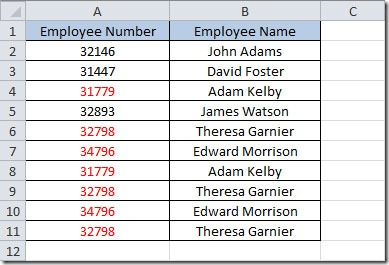On cell A2, I created a Conditional Formatting with a condition "Formula is" and putted the function =COUNTIF(A:A,A2)>1. The COUNTIF function has the following syntax:

COUNTIF(range,criteria)

range is the area where you you want to check if it finds the value specified by criteria.

This will return TRUE if the value from cell A2 is found more than 1 time in column A. If this is TRUE it will apply the Format that I defined. I then Copy and Paste Special-Format to the rest of the cells in column A.

In Excel 2007 and 2010, you can use the a new option on the Conditional Formatting menu that is the Duplicate Values.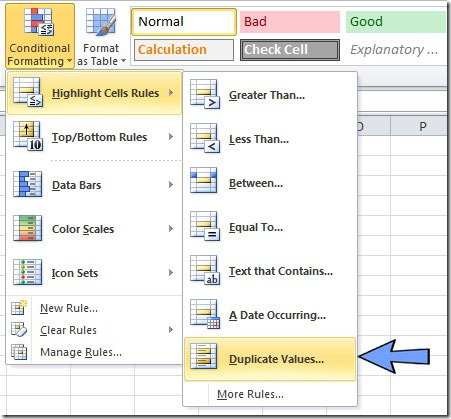Just select the range where you want to highlight the duplicate and go to the menu bar and choose Highlight Cells Rules-Duplicate Values. A Duplicate Values dialog box will open. You can choose to highlight the Duplicate or the Unique values and you can set the color that you want to use to highlight the values.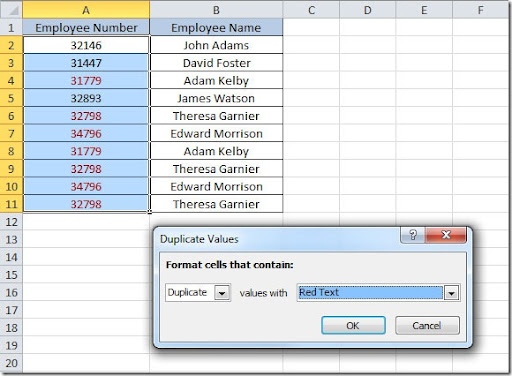The result will be the same as using the COUNTIF solution. On Excel 2003 you don’t have this Duplicate Values option on the Conditional Formatting menu.

### IF() – Checking conditions

The IF() function is one of the most used in Excel. It’s very simple to use. The syntax for this function is:

IF(logical_test,value_if_true,value_if_false)

logical_test is the value or expression that we want to evaluate. value_if_true is the result if the condition of logical_test is true and value_if_false is the result if the condition is false.

Examples:

=IF(A2=100,"True","False") will check if there is a value 100 in cell A2. If true, will put on the cell where we have the formula, the value “True”. If the value in cell A2 it’s not 100 the formula will return “False” on the cell.

=IF(AND(A2=100,A3=200),"True","False") this is the same as the last example with the exception that in this case we are checking two condition and only if both are true, the result will be “True”.

### VBA – Copy all Worksheets to another Workbook

If you want to copy all of your worksheets from the actual workbook to another workbook, you can use this simple VBA code to do it.

Sub copy_sheets()

Dim wb1 As Workbook
Dim wb2 As Workbook
wb1 = ActiveWorkbook
'Change the name of the destination workbook here
wb2 = Workbooks("Destination.xls")
For Each Sheet In wb1.Sheets
If Sheet.Visible = True Then
'copy the sheets after the last
'sheet of the destination workbook
Sheet.Copy After:=wb2.Sheets(wb2.Sheets.Count)
End If
Next Sheet

End Sub

### DATEVALUE() - Convert text values to dates

Sometimes we have values on our sheets that are stored as text (strings) and we need to convert them to date format. For this we can use the DATEVALUE() function. The syntax for this function is:

DATEVALUE(date_text)

date_text argument is a text string.

This function can convert various types of strings. Here are some samples:

DATEVALUE("15MAR") will return 15-03-2009
DATEVALUE("MAR09") will return 01-03-2009
DATEVALUE("15/3") will return 15-03-2009
DATEVALUE("1-MAR") will return 01-03-2009

### Replace value on several Excel files

I’ve written this code for a user that wanted to change a value in hundreds of Excel files that where in several sub folders under a main folder. This code will go through the main folder C:\Test and will replace the value on cell A1 on Sheet1 of every Excel file it will find. You can adapt this code by changing the folder name, cell destination or sheet name.

Sub Change_Value_On_Files()

Dim fso, folder, files, NewsFile, sFolderSet
objExcel = CreateObject("Excel.Application")
objExcel.Visible = TrueSet
fso = CreateObject("Scripting.FileSystemObject")
sFolder = "C:\Test"
Set folder = fso.GetFolder(sFolder)
Set files = folder.files
For Each SubFolder In folder.SubFolders
For Each folderIdx In SubFolder.files
If Right(folderIdx, 4) = ".xls" Then
Set objWorkbook = objExcel.Workbooks.Open(folderIdx)
Set objWorksheet = objWorkbook.Worksheets(1)
Set objRange = objWorksheet.Range("A1")
objWorkbook.Save
objWorkbook.Close True
End If
Next
Next

End Sub

### VLOOKUP() - Get value based on another value

If we have a table of data, like the one shown bellow, and based on a value of that table want to return the value from another column we can use the VLOOKUP() function.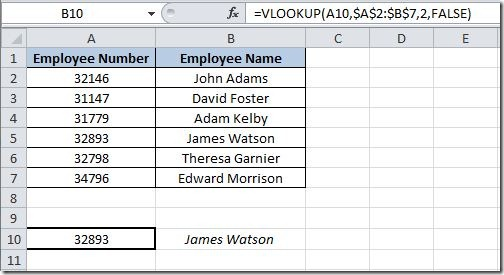The syntax for this function is:

VLOOKUP(lookup_value,table_array,col_index_num,range_lookup)

In our example, on cell B10 I've putted this formula:

=VLOOKUP(A10,\$A\$2:\$B\$7,2,FALSE)

First we have the lookup value that is the value that we want to check is it's on the table. In this example is the value on cell A10.

Then we have the table array. This is the range of cells where we want to check for the value on cell A10. In this case, we want to lookup the value on range A2:B7. If it finds the value of A10, it will the return the value referred by the col_index_num argument of the function. In this case we want to return the value from column 2 (the employee name).

The range_lookup argument is optional and it can have TRUE or FALSE value. If you put TRUE or omit this argument, it will return an approximated match. If it doesn't find the value of the lookup_value it will return the next largest value that is less than the lookup_value. If you put FALSE, it will find an exact match and if it doesn't find one it will return the error value #N/A.

So, on this example, we want to find the value 32893 and get the corresponding value from column 2, in this case the returned value will be James Watson.

If the value specified on the lookup_value is not found, and the range_lookup is FALSE, then the formula will return a #N/A error value. We can change our formula so that it doesn't display this error with this formula:

=IF(ISNA(VLOOKUP(A10,\$A\$2:\$B\$7,2,FALSE)),"",VLOOKUP(A10,\$A\$2:\$B\$7,2,FALSE))

In this case we use two more functions, the IF and ISNA. I will not explain the IF function in this article, will handle this function on a separated article on the future.

The ISNA() function checks if the VLOOKUP() function returns a #N/A error value. So this formula will check if the VLOOKUP() function returns #N/A. If it does, the cell will be empty. If it doesn't it will show the result of the VLOOKUP function.

### SUMPRODUCT() - Sum values based on conditions

Sometimes we want to sum values from a table based on conditions that we input into another cell. Take a look at the table below. Here we have a table of weekly values for each vendor.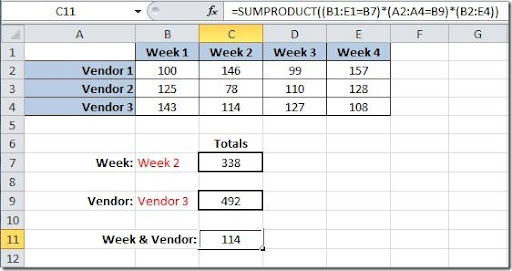In this case we want to input on cell B7 the week value and get the total for this week on cell C7. This can be done using the SUMPRODUCT() function. The syntax for this function is like this:

SUMPRODUCT(array1,array2,…) you can have up to 30 arrays.

The formula in C7 for this will:

=SUMPRODUCT((B1:E1=B7)*(B2:E4))

Checks on range B1:E1 for the value in cell B7 and sums the values under that value. On this example the value "Week 2" can be found on C1 so the sum will be done with the values from C2:C4 and the result will be 338.

Another example is putting in B9 the vendor name and get the total for this vendor on cell C9. The formula will be like this:

=SUMPRODUCT((A2:A4=B9)*(B2:E4))

Checks on range A2:A4 for the value in cell B9 and sums the values for that row where it finds the vendor. On this example the vendor "Vendor 3" can be found on row 4 so the sum will be done with the range B4:E4 and the result will be 492.

Finally we want to get the value for Week 2 for Vendor 3. We can use also the SUMPRODUCT function like this:

=SUMPRODUCT((B1:E1=B7)*(A2:A4=B9)*(B2:E4))

It will return the value 114 that corresponds to Vendor 3 (row 4) and Week 2 (column C).

These are simple examples of how you can use the SUMPRODUCT function. It can be used in more complex formulas that I will address later on another article.

I live in Setúbal, Portugal. I work with Excel for more than 10 years. This tool is so powerful and complete that I'm still learning to work with it. Everyday I discover new fixtures and ways of doing things in Excel.
I'm an active member of the Experts Exchange Community forums. where I’ve more than 1,600 questions answered. I try to help others solve their problems in Excel and also in other tools, mainly Microsoft tools (Access, Outlook, Visual Basic .Net, Powerpoint).
I'm also a Microsoft Office Specialist. I'm a self-taught person but I'm also taking a degree in Information Systems Management.
I started writing this blog to share with others what I've learned over the years that I use Excel and with the experience in problem solving that I've got from my participation on Excel forums. Hope that you recognize the work I've done here.

Several articles published on other websites:
Microsoft Excel Blog:

Pplware (Tech page from SAPO Portuguese portal – articles in PT):

VLOOKUP Week:

Programar Magazine (article in PT):

Several tips & tricks videos published on Microsoft knowledge sharing site:
120segundos.net (Videos in PT): http://120segundos.net/videos/pesquisa?q=excel

### Contacts

You can contact me by email at: jpgpinto (at) gmail (dot) com

Would like to hear from you. If you have some comments or sugestions about this blog, feel free to send me an email. I'll try to answer as soon as possible.

### Consulting

I'm available to do consultant work. Depending on your project, I can take a look and provide a custom quote for it. Each project is a challenge, so I don't work with a fixed fee. I can handle, for instance:

- Worksheets/ workbooks improvement;
- Custom applications development;
- User forms development;
- Excel Integration with other Office programs (Outlook, Powerpoint, etc)
- VBA features to automate your work;
- Worksheet problem solving;
- Excel Training.

You can contact me by email at: jpgpinto (at) gmail (dot) com. Drop me an email and I will find the solution for your problem.